International
Tables for
Crystallography
Volume B
Reciprocal space
Edited by U. Shmueli

International Tables for Crystallography (2010). Vol. B, ch. 1.3, pp. 51-52   | 1 | 2 |

## Section 1.3.2.7.5. Properties of the discrete Fourier transform

G. Bricognea

aGlobal Phasing Ltd, Sheraton House, Suites 14–16, Castle Park, Cambridge CB3 0AX, England, and LURE, Bâtiment 209D, Université Paris-Sud, 91405 Orsay, France

#### 1.3.2.7.5. Properties of the discrete Fourier transform

| top | pdf |

The DFT inherits most of the properties of the Fourier transforms, but with certain numerical factors (Jacobians') due to the transition from continuous to discrete measure.

 (1) Linearity is obvious. (2) Shift property. Ifand, where subtraction takes place by modular vector arithmetic inand, respectively, then the following identities hold:(3) Differentiation identities. Let vectors ψ and Ψ be constr­ucted fromas in Section 1.3.2.7.3, hence be related by the DFT. Ifdesignates the vector of sample values ofat the points of, andthe vector of values ofat points of, then for all multi-indicesor equivalently(4) Convolution property. Letand(respectively ψ and Ψ) be related by the DFT, and defineThen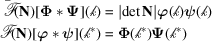and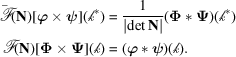Since addition onandis modular, this type of convolution is called cyclic convolution. (5) Parseval/Plancherel property. If ϕ, ψ, Φ, Ψ are as above, then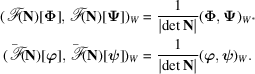(6) Period 4. When N is symmetric, so that the ranges of indicesandcan be identified, it makes sense to speak of powers ofand. Then the standardized' matrices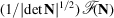and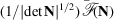are unitary matrices whose fourth power is the identity matrix (Section 1.3.2.4.3.4); their eigenvalues are thereforeand.